# Time-frequency analysis of combined MEG/EEG data

## Introduction

In this tutorial you can find information about the time-frequency analysis of a single subject’s EEG-MEG data using a Hanning window. This tutorial also shows how to visualize the results, which will now have an extra dimension beyond time and sensor: frequency. We will pay special attention to differences between EEG and MEG, which will shown themselves not only in visualizing the results, but also in the effects of having a reference in EEG, i.e. of having relative signals versus absolute signals in MEG. We will also compare conditions in the frequency domain, looking at differences in beta-rebound after left versus the right hand responses. Familiarize yourself with the paradigm and data we recorded by re-reading the example dataset description

This tutorial contains the hands-on material of the NatMEG workshop and is complemented by this lecture.

## Background

Oscillatory components contained in the ongoing EEG or MEG signal often show power changes relative to experimental events. These signals are not necessarily phase-locked to the event and will not be represented in event-related fields and potentials (Tallon-Baudry and Bertrand (1999)). The goal of this section is to compute and visualize event-related changes by calculating time-frequency representations (TFRs) of power. This will be done using analysis based on Fourier analysis and wavelets. The Fourier analysis will include the application of multitapers (Mitra and Pesaran (1999), Percival and Walden (1993)) which allow a better control of time and frequency smoothing.

Calculating time-frequency representations of power is done using a sliding time window. This can be done according to two principles: either the time window has a fixed length independent of frequency, or the time window decreases in length with increased frequency. For each time window the power is calculated. Prior to calculating the power one or more tapers are multiplied with the data. The aim of the tapers is to reduce spectral leakage and control the frequency smoothing.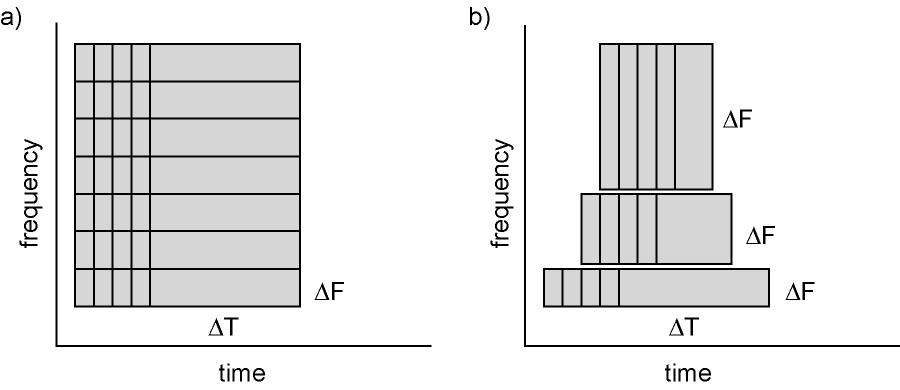Figure: Time and frequency smoothing. (a) For a fixed length time window the time and frequency smoothing remains fixed. (b) For time windows that decrease with frequency, the temporal smoothing decreases and the frequency smoothing increases.

If you want to know more about tapers/window functions you can have a look at this Wikipedia site. Note that Hann window is another name for Hanning window used in this tutorial. There is also a Wikipedia site about multitapers, to take a look at it click here. In this tutorial we will only look at Hanning tapers, and spend our time more in noticing the similarities and differences between MEG and EEG.

## Procedure

To calculate the time-frequency analysis for the example dataset we will perform the following steps for both MEG and EE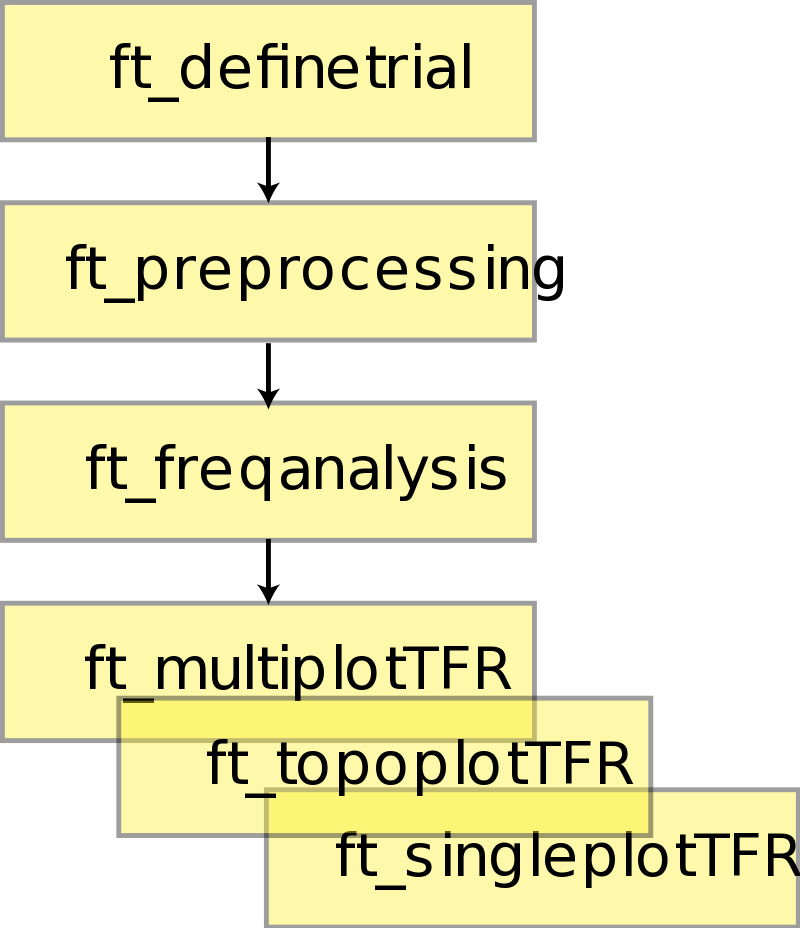Figure: Schematic overview of the steps in time-frequency analysis

## Preprocessing MEG

The first step is to read the data using the function ft_preprocessing. With the aim to reduce boundary effects occurring at the start and the end of the trials, it is recommended to read larger time intervals than the time period of interest. In this example, the time of interest is from -1.0 s to 1.5 s (t = 0 s defines the time of response); however, the script reads the data from -1.5 s to 2.0 s.

As with the previous preprocessing tutorial, we will preprocess the MEG and EEG data separately. We will start with MEG magnetometers, then move to EEG before looking at the planar gradiometers in MEG.

The MEG dataset that we use in this tutorial is available as oddball1_mc_downsampled.fif from our download server. Furthermore, you should download and save the custom trial function trialfun_oddball_responselocked.m to a directory that is on your MATLAB path.

``````cfg = [];
cfg.dataset = 'oddball1_mc_downsampled.fif';
cfg.channel = 'MEG';

% define trials based on responses
cfg.trialdef.prestim       = 1.5;
cfg.trialdef.poststim      = 2.0;
cfg.trialdef.stim_triggers = [1 2];
cfg.trialdef.rsp_triggers  = [256 4096];
cfg.trialfun               = 'trialfun_oddball_responselocked';
cfg                        = ft_definetrial(cfg);

% preprocess MEG data
cfg.continuous             = 'yes';
cfg.demean                 = 'yes';
cfg.dftfilter              = 'yes';
cfg.dftfreq                = [50 100];

data_MEG_responselocked    = ft_preprocessing(cfg);

% write data to disk
save data_MEG_responselocked data_MEG_responselocked -v7.3
``````

### Clean data

At this stage in the processing pipeline you could remove bad trials using, for example, ft_rejectvisual. We are going to skip this for now as we do not have a lot of trials for this part of the analysis and the data is relatively clean. Furthermore, be aware that removing trials in this way could create a bias towards removing more trials in one condition than in the other due to differences in variance between the conditions.

## Time-frequency analysis with a Hanning taper and fixed window length

We will here describe how to calculate time frequency representations using Hanning tapers. When choosing for a fixed window length procedure the frequency resolution is defined according to the length of the time window (delta T). The frequency resolution (delta f in the first) = 1/length of time window in sec (delta T in the first figure). Thus a 500 ms time window results in a 2 Hz frequency resolution (1/0.5 sec= 2 Hz) meaning that power can be calculated for 2 Hz, 4 Hz, 6 Hz etc. An integer number of cycles must fit in the time window. In the following example a time window with length 500 ms is applied.

Since we have two conditions (responses with left and right index finger), we will calculate the data separately for both so that we can compare them. We select the trials based on the .trialinfo field. We created this field when we called trialfun_oddball_responselocked in ft_definetrial. In addition to the three colums in the .trl, it also added a column with response side based on the response trigger (256 and 2048 for left and right, respectively). After preprocessing, this column is added in the data structure as the field .trialinfo. This is a good example of keeping your own internal bookkeeping. You can e.g., also add response times, or accuracy. This info will travel with you throughout your analysis as long as it represents separate trials (and not averages).

``````cfg              = [];
cfg.output       = 'pow';
cfg.channel      = 'all';
cfg.method       = 'mtmconvol';
cfg.taper        = 'hanning';
cfg.toi          = [-1 : 0.10 : 1.5];
cfg.foi          = 1:40;
cfg.t_ftimwin    = ones(size(cfg.foi)) * 0.5;

cfg.trials       = find(data_MEG_responselocked.trialinfo(:,1) == 256);
TFR_left_MEG     = ft_freqanalysis(cfg, data_MEG_responselocked);

cfg.trials       = find(data_MEG_responselocked.trialinfo(:,1) == 4096);
TFR_right_MEG    = ft_freqanalysis(cfg, data_MEG_responselocked);

% save data
save TFR_left_MEG TFR_left_MEG
save TFR_right_MEG TFR_right_MEG
``````

Regardless of the method used for calculating the TFR, the output format is identical. It is a structure with the following element

``````TFR_left_MEG =

label: {464x1 cell}         % Channel names
dimord: 'chan_freq_time'     % Dimensions contained in powspctrm, channels X frequencies X time
freq: [1x40 double]        % Array of frequencies of interest (the elements of freq may be different from your cfg.foi input depending on your trial length)
time: [1x26 double]        % Array of time points considered
powspctrm: [464x40x26 double]   % 3-D matrix containing the power values
elec: [1x1 struct]         % Electrode positions etc `<fixme>`
cfg: [1x1 struct]         % Settings used in computing this frequency decomposition
``````

The field TFR_left_MEG.powspctrm contains the temporal evolution of the raw power values for each specified frequency in the left response conditions.

## Visualization

This part of the tutorial shows how to visualize the results of any type of time-frequency analysis.

To visualize the event-related power changes, a normalization with respect to a baseline interval will be performed. There are two possibilities for normalizing:

• Subtracting, for each frequency, the average power in a baseline interval from all other power values. This gives, for each frequency, the absolute change in power with respect to the baseline interval.
• Expressing, for each frequency, the raw power values as the relative increase or decrease with respect to the power in the baseline interval. This means active period/baseline. Note that the relative baseline is expressed as a ratio; i.e. no change is represented by 1.

There are three ways of graphically representing the data: 1) time-frequency plots of all channels, in a quasi-topographical layout, 2) time-frequency plot of an individual channel, 3) topographical 2-D map of the power changes in a specified time-frequency interval.

``````cfg = [];
cfg.baseline     = [-0.5 -0.1];
cfg.baselinetype = 'absolute';
cfg.zlim         = [-2e-26 2e-26];
cfg.showlabels   = 'yes';
cfg.layout       = 'neuromag306mag.lay';
cfg.channel      = 'MEG*1';

figure;
ft_multiplotTFR(cfg, TFR_left_MEG);
print -dpng natmeg_freq3.png
``````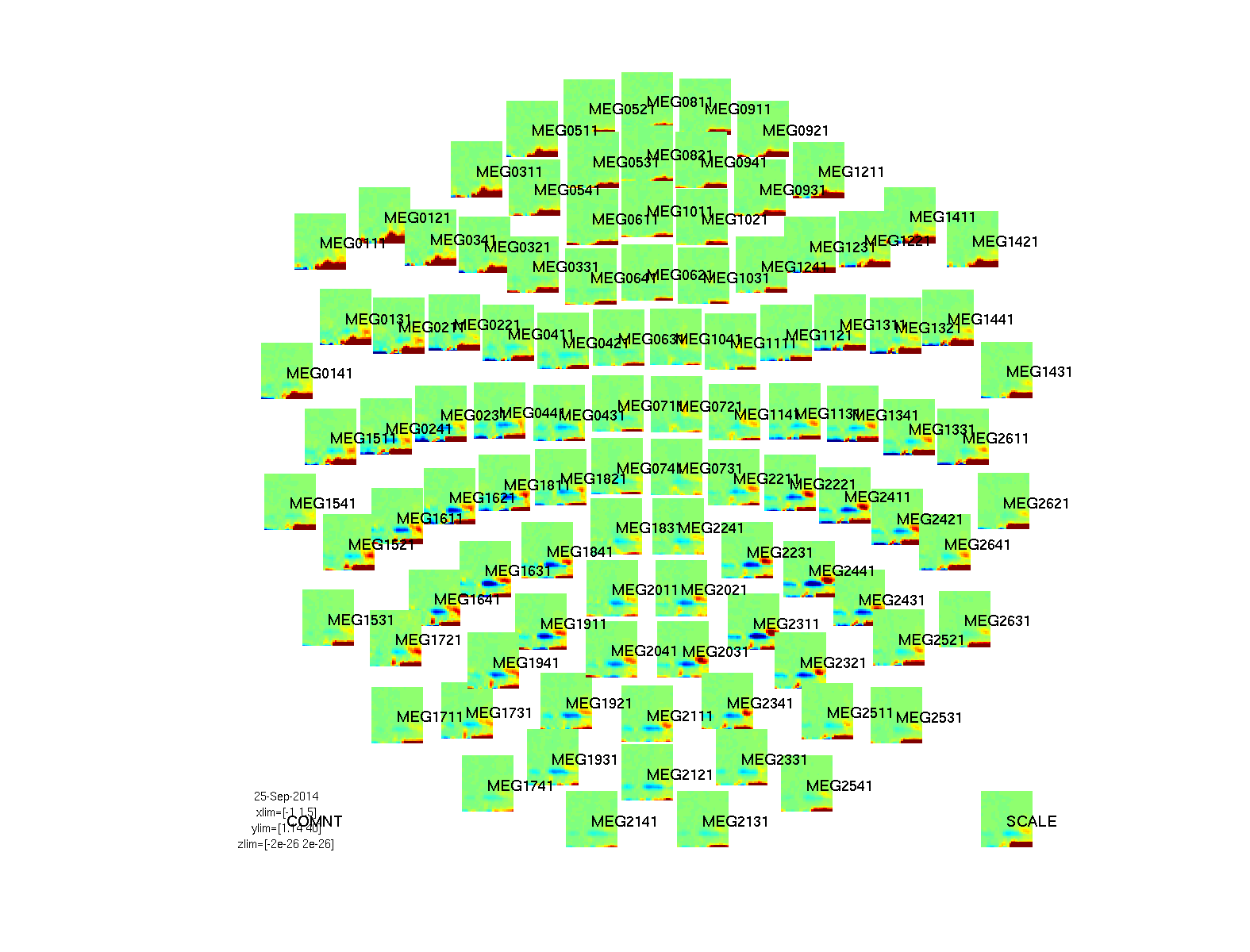Figure: Time-frequency representations calculated using ft_freqanalysis and plotted with ft_multiplotTFR

Note that by using the options cfg.baseline and cfg.baselinetype when calling plotting functions, baseline correction can be applied to the data. Baseline correction can also be applied directly by calling ft_freqbaseline. You can combine the various visualization options/functions interactively to explore your data. Currently, this is the default plotting behavior because the configuration option cfg.interactive=’yes’ is activated unless you explicitly select cfg.interactive=’no’ before calling ft_multiplotTFR to deactivate it. See also the plotting tutorial for more details.

Something interesting seems to happen at channel MEG1041. To make a plot of a single channel use the function ft_singleplotTFR.

``````cfg = [];
cfg.baseline     = [-0.5 -0.1];
cfg.baselinetype = 'absolute';
cfg.zlim         = [-1e-26 1e-26];
cfg.channel      = 'MEG1041';

figure;
ft_singleplotTFR(cfg, TFR_left_MEG);
print -dpng natmeg_freq4.png
``````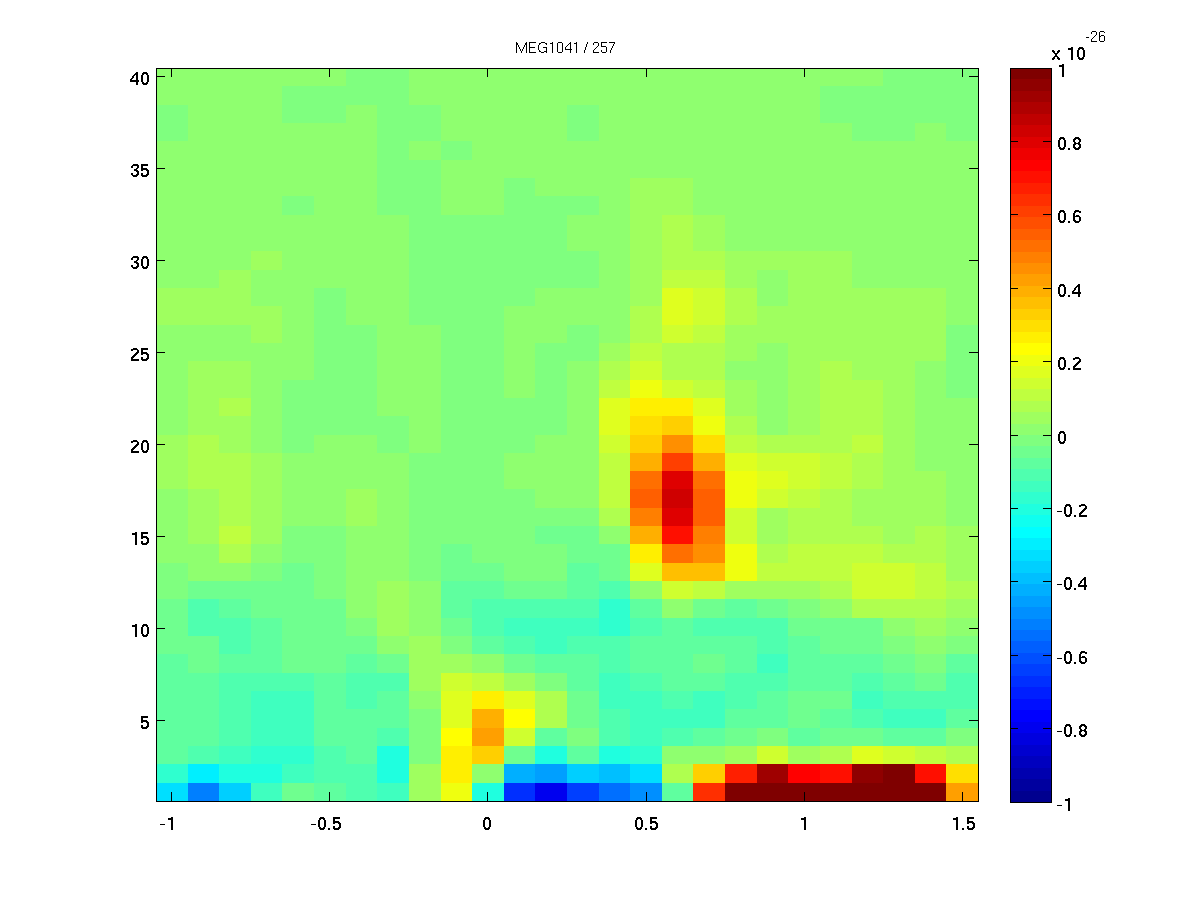Figure: The time-frequency representation for a single sensor, obtained using ft_singleplotTFR

From the previous figure you can see that there is an increase in power around 15-25 Hz in the time interval 0.4 to about 0.8 s after response onset. To show the topography of the beta increase use the function ft_topoplotTFR.

``````cfg = [];
cfg.baseline     = [-0.5 -0.1];
cfg.baselinetype = 'absolute';
cfg.xlim         = [0.4 0.8];
cfg.zlim         = [-4e-27 4e-27];
cfg.ylim         = [15 25];
cfg.marker       = 'on';
cfg.layout       = 'neuromag306mag.lay';
cfg.channel      = 'MEG*1';

figure;
ft_topoplotTFR(cfg, TFR_left_MEG);
print -dpng natmeg_freq5.png
``````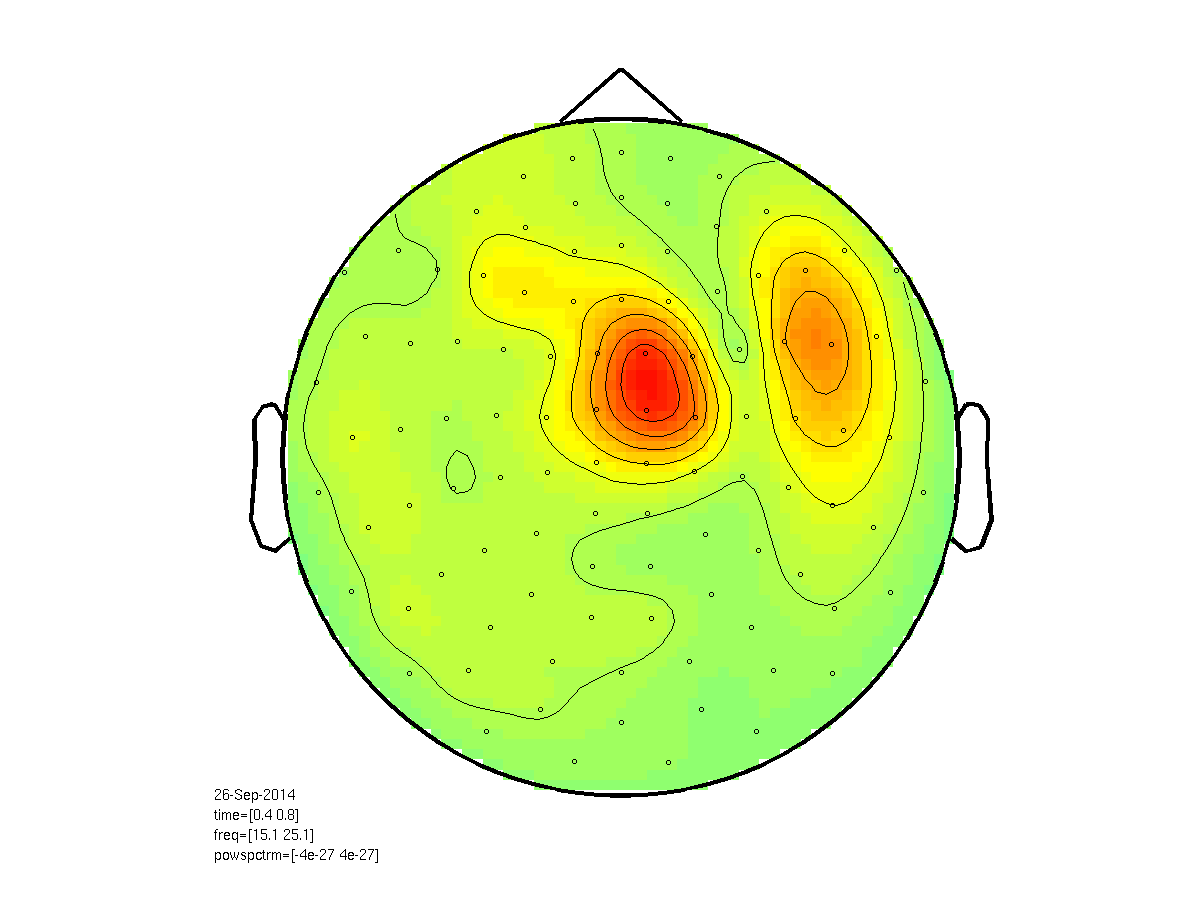Figure: Topographic representation of the time-frequency representations of beta (15-25 Hz) after (0.5-1.0s) left-finger response, obtained using ft_topoplotTFR.

So what do you think we are looking at? I guess the introduction gave it away, but how would you argue for it?

Perhaps we should now also look at the beta-rebound after a response of the other (right) hand? We can use the same parameters to make them comparable

``````cfg = [];
cfg.baseline     = [-0.5 -0.1];
cfg.baselinetype = 'absolute';
cfg.xlim         = [0.4 0.8];
cfg.zlim         = [-4e-27 4e-27];
cfg.ylim         = [15 25];
cfg.marker       = 'on';
cfg.layout       = 'neuromag306mag.lay';
cfg.channel      = 'MEG*1';

figure;
ft_topoplotTFR(cfg, TFR_right_MEG);
print -dpng natmeg_freq6.png
``````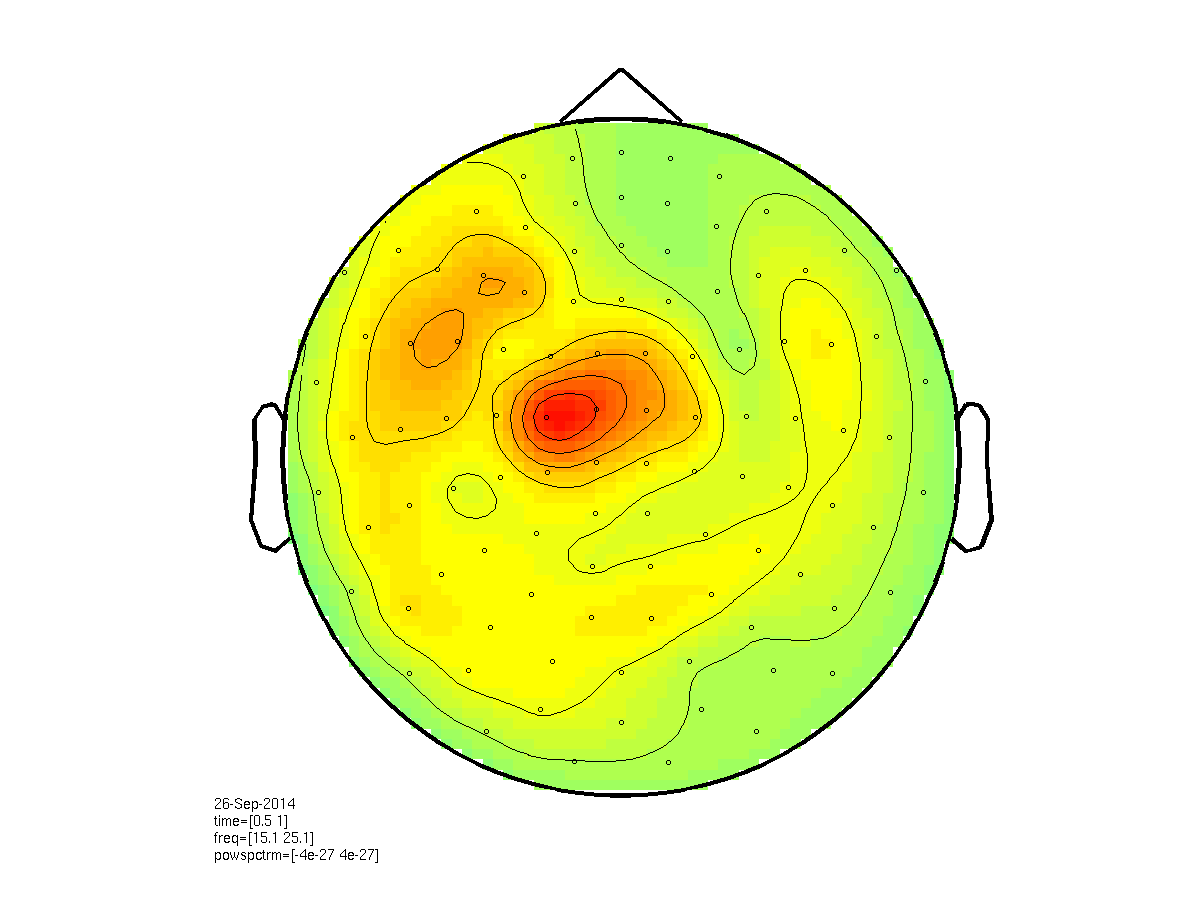Figure: Topographic representation of the time-frequency representations of beta (15-25 Hz) after (0.5-1.0s) right-finger response, obtained using ft_topoplotTFR

Until now we have been using an (absolute) baseline. However, because we have two conditions with an - assumed - similar baseline, we can also compare the two conditions directly by subtracting and then dividing the two powerspectra by there sum, thereby normalizing by their common activity using ft_math.

``````cfg = [];
cfg.parameter = 'powspctrm';
cfg.operation = '(x1-x2)/(x1+x2)';

TFR_diff_MEG = ft_math(cfg, TFR_right_MEG, TFR_left_MEG);

cfg = [];
cfg.xlim         = [0.4 0.8];
cfg.zlim         = [-0.4 0.4];
cfg.ylim         = [15 25];
cfg.marker       = 'on';
cfg.layout       = 'neuromag306mag.lay';
cfg.channel      = 'MEG*1';

figure;
ft_topoplotTFR(cfg, TFR_diff_MEG);
print -dpng natmeg_freq7.png
``````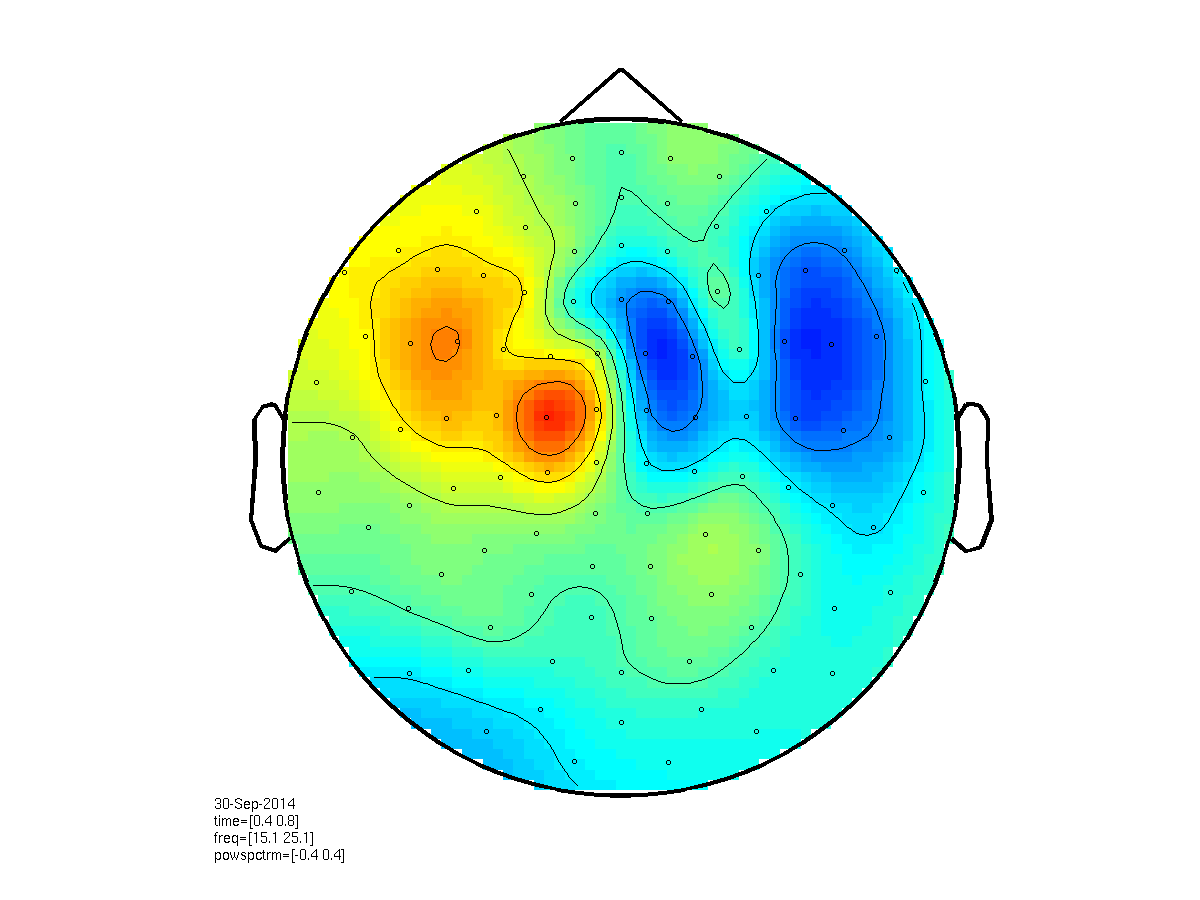Figure: Topographic representation of the time-frequency representations of the difference in beta (15-25 Hz) power, between left and right response, after 0.5-1.0s, obtained using ft_topoplotTFR.

## Preprocessing EEG

We will now proceed with doing the time-frequency analysis of the EEG data. Note we are using mainly similar parameters as in the MEG analysis.

Keep an eye open for the differences in processing and visualizing EEG.

``````cfg = [];
cfg.dataset = 'oddball1_mc_downsampled.fif';

% define trials based on responses
cfg.trialdef.prestim       = 1.5;
cfg.trialdef.poststim      = 2.0;
cfg.trialdef.stim_triggers = [1 2];
cfg.trialdef.rsp_triggers  = [256 4096];
cfg.trialfun               = 'trialfun_oddball_responselocked';
cfg                        = ft_definetrial(cfg);

% preprocess EEG data
cfg.channel                = 'EEG';
cfg.continuous             = 'yes';
cfg.demean                 = 'yes';
cfg.dftfilter              = 'yes';
cfg.dftfreq                = [50 100];

data_EEG_responselocked    = ft_preprocessing(cfg);

% save the data
save data_EEG_responselocked data_EEG_responselocked -v7.3
``````

### Clean data

As with the MEG data we are going to skip trial rejection. However, if you wish to check for bad trials you can do so using, for example, ft_rejectvisual.

Especially EEG records can contain bad channels due to various reasons such as bad impedance, broken channels, etc. In the next steps we will therefor use ft_rejectvisual to select bad channels. We will then fix these channels by replacing the values of these channels with an average of its neighbours. Note that we have not re-referenced our data yet. We should remove bad channels prior to re-referencing or the noise from these channels will be present in all channels.

``````% select bad channels
cfg = [];
cfg.metric  = 'var';
temp        = ft_rejectvisual(cfg, data_EEG_responselocked);

% with this little trick we get the names of the selected channels

clear temp
``````

Fixing bad channels is usually done by interpolating between neighbouring channels. Therefore we have to determine which channels are neighbours. Using the information of the position of the electrodes and some mathematics we can calculate which channels are neighbours of each othe

``````% determine neighbours structure
cfg            = [];
cfg.method     = 'triangulation';
cfg.senstype   = 'EEG'; % Our data still contains information from the MEG channels, we want to make sure ft_prepare_neighbours does not get confused
neighbours_EEG = ft_prepare_neighbours(cfg, data_EEG_responselocked);

% plotting neighbours
cfg            = [];
cfg.neighbours = neighbours_EEG;
cfg.senstype   = 'EEG';
ft_neighbourplot(cfg, data_EEG_responselocked);
print -dpng natmeg_freq8.png
``````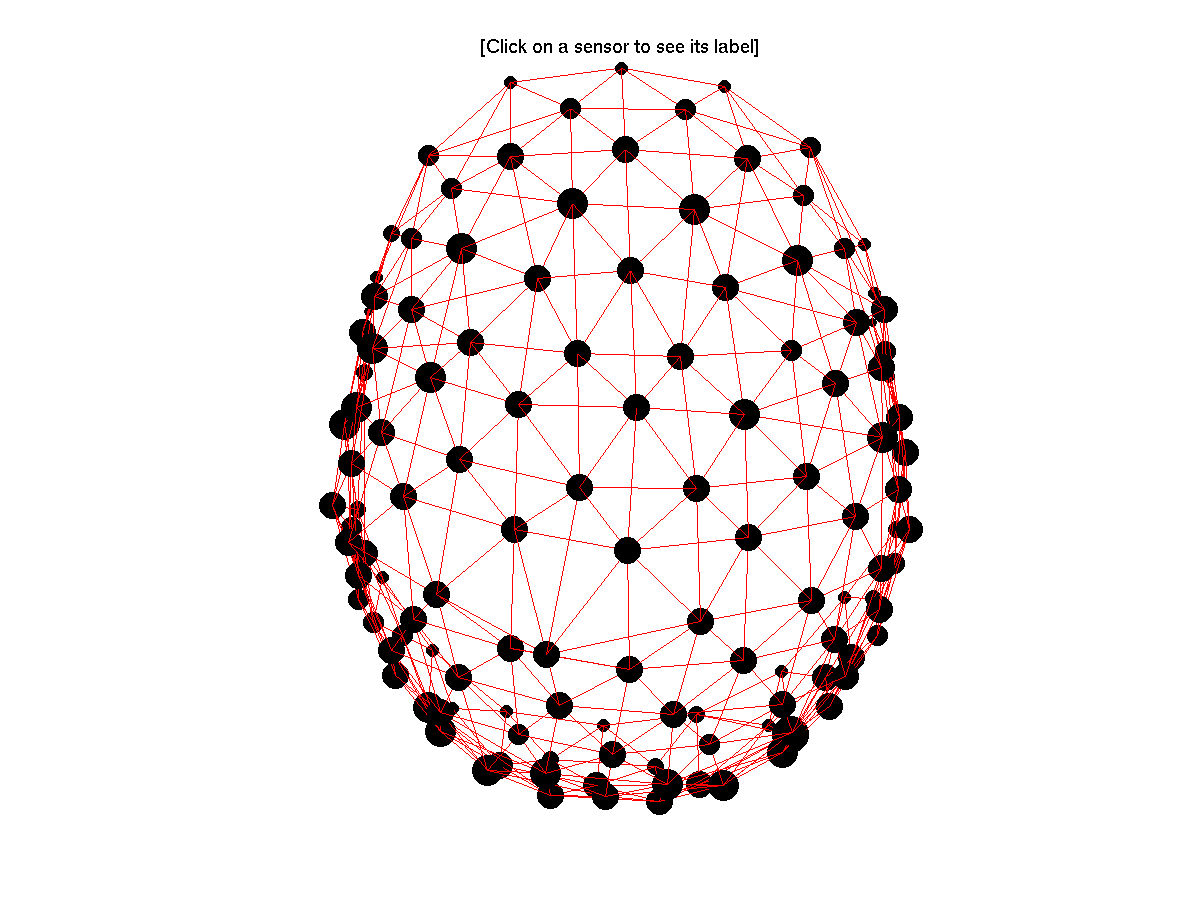Figure: 3-D representation of the neighbourstructure, obtained using ft_neighbourplot.

We can then use this neighborhood structure to fix our bad channels with ft_channelrepair, by replacing their values with interpolations between their neighbours.

``````% fix channels
cfg = [];
cfg.method                    = 'spline';
cfg.neighbours                = neighbours_EEG;
cfg.senstype                  = 'EEG';
data_clean_EEG_responselocked = ft_channelrepair(cfg, data_EEG_responselocked);

% save the data
save data_clean_EEG_responselocked data_clean_EEG_responselocked
``````

Now that we have repaired our bad channels we can re-reference our data to the common average.

``````cfg = [];
cfg.reref                  = 'yes';
cfg.refchannel             = 'all';

data_clean_EEG_responselocked = ft_preprocessing(cfg, data_clean_EEG_responselocked);
``````

## Time-frequency analysis with a Hanning taper and fixed window length

We are now ready to do the same frequency analysis on EEG as we did on MEG. Again, separately for left and right response.

``````cfg              = [];
cfg.output       = 'pow';
cfg.channel      = 'all';
cfg.method       = 'mtmconvol';
cfg.taper        = 'hanning';
cfg.toi          = [-1 : 0.10 : 1.5];
cfg.foi          = 1:40;
cfg.t_ftimwin    = ones(size(cfg.foi)) * 0.5;

cfg.trials       = find(data_clean_EEG_responselocked .trialinfo(:,1) == 256);
TFR_left_EEG     = ft_freqanalysis(cfg, data_clean_EEG_responselocked );

cfg.trials       = find(data_clean_EEG_responselocked .trialinfo(:,1) == 4096);
TFR_right_EEG    = ft_freqanalysis(cfg, data_clean_EEG_responselocked );

% save data
save TFR_left_EEG TFR_left_EEG
save TFR_right_EEG TFR_right_EEG
``````

Great! Now lets plot the EEG and see what we get.

``````cfg = [];
cfg.baseline     = [-0.5 -0.1];
cfg.baselinetype = 'absolute';
cfg.xlim         = [0.5 1.0];
cfg.zlim         = [-4e-12 4e-12];
cfg.ylim         = [15 25];
cfg.marker       = 'on';
cfg.layout       = 'natmeg_customized_eeg1005.lay';

figure;
ft_topoplotTFR(cfg, TFR_left_EEG);
print -dpng natmeg_freq9.png
``````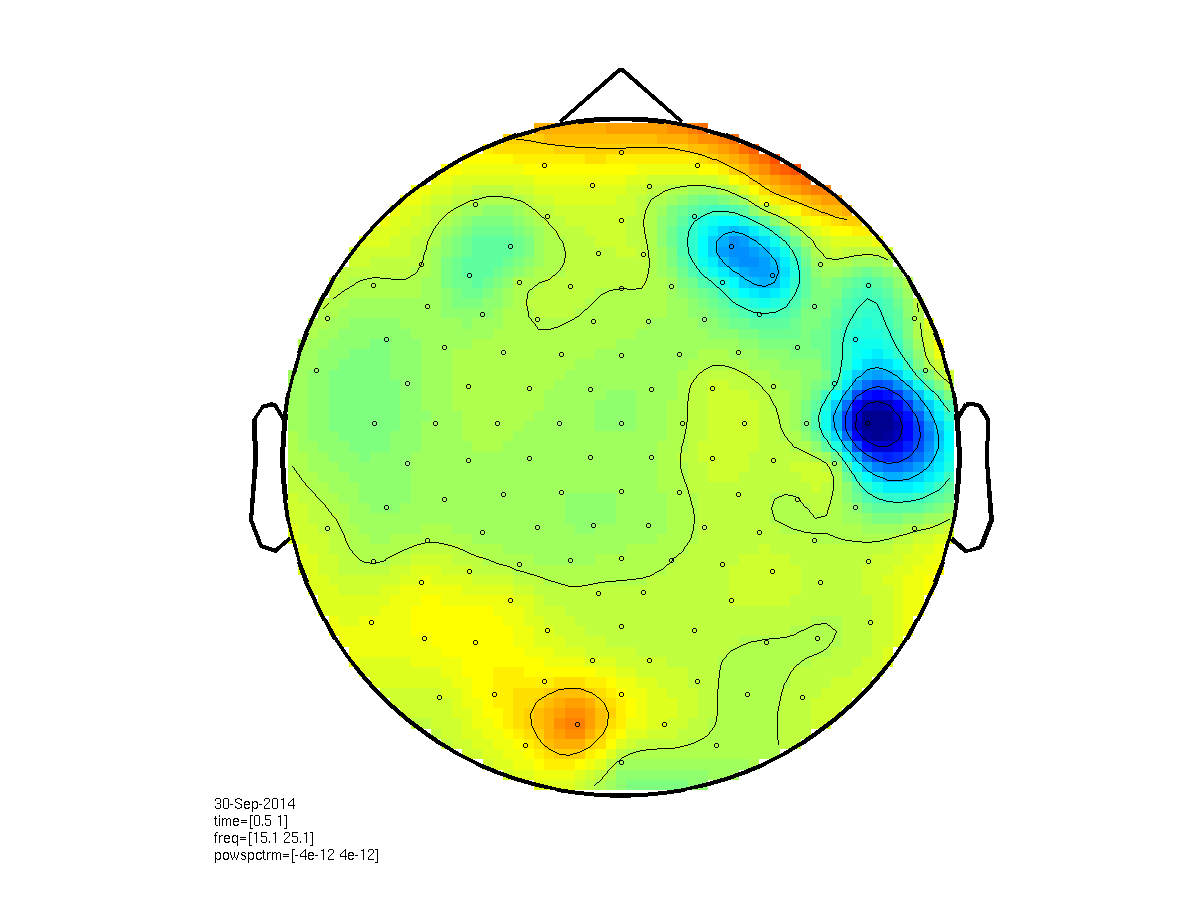Figure: Frequency topography (EEG) calculated using ft_freqanalysis. Plotting was done with ft_topoplotTFR.

Hmmm… What do you think? A bit strange, right? Is this real, or perhaps an artifact? Try to find it out using the interactive mode.

In fact, we are now encountering an aspect of EEG recordings we haven’t seen in MEG before. Take a look what happens when instead of an absolute baseline we use a relative baseline.

``````cfg = [];
cfg.baseline     = [-0.5 -0.1];
cfg.baselinetype = 'relchange';
cfg.ylim         = [15 25];
cfg.xlim         = [0.5 1.0];
cfg.zlim         = [-1.2 1.2];
cfg.layout       = 'natmeg_customized_eeg1005.lay';

figure;
ft_topoplotTFR(cfg, TFR_left_EEG);
print -dpng natmeg_freq10.png
``````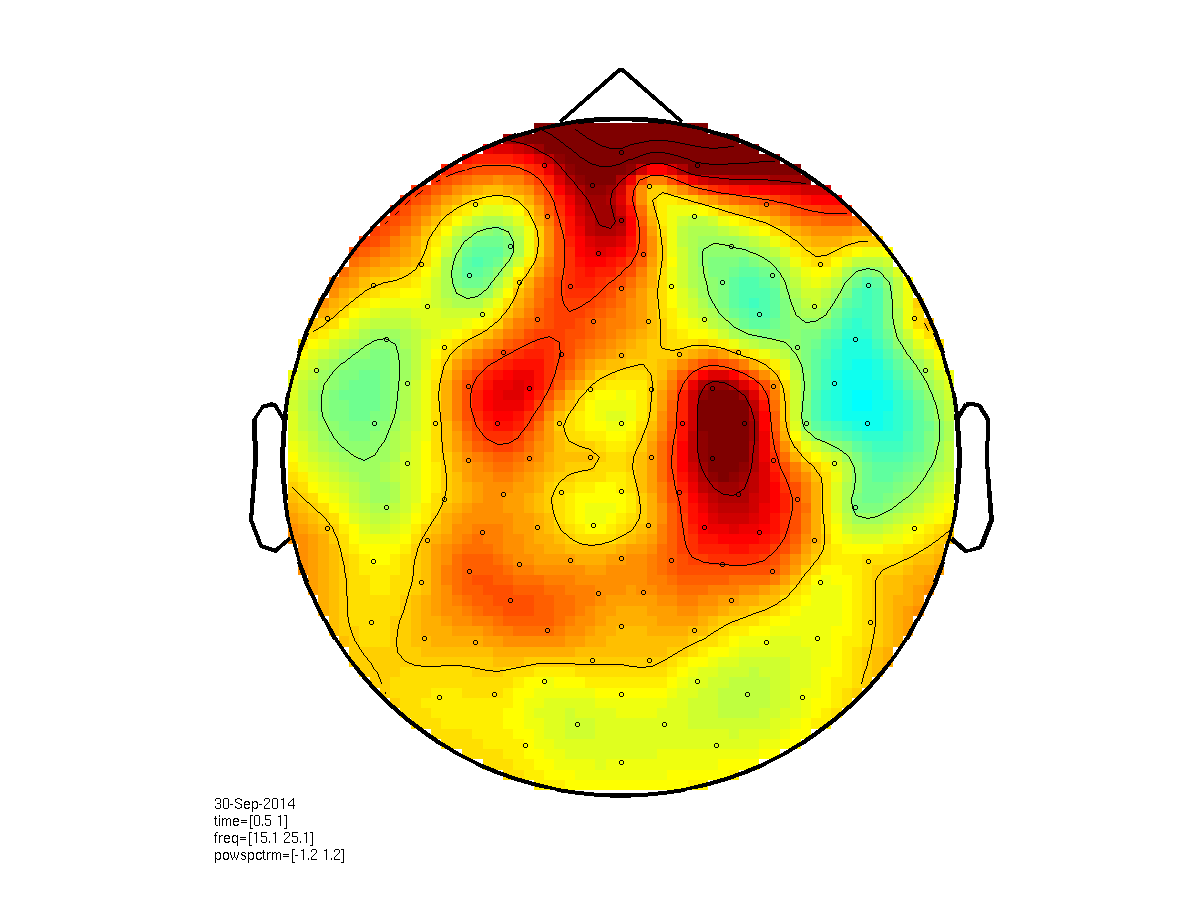Figure: Frequency topography (EEG) calculated using ft_freqanalysis. Plotting was done with ft_topoplotTFR.

Why this difference?

To round up our comparison between EEG and MEG, lets plot the difference between conditions.

This will at the same time solved the above problem. Can you say why?

``````cfg = [];
cfg.parameter    = 'powspctrm';
cfg.operation    = '(x1-x2)/(x1+x2)';

TFR_diff_EEG = ft_math(cfg, TFR_right_EEG, TFR_left_EEG);

% if ft_math didn't work, then just do it by hand - its exactly the sam
TFR_diff_EEG = TFR_right_EEG;
TFR_diff_EEG.powspctrm = (TFR_right_EEG.powspctrm - TFR_left_EEG.powspctrm) ./ (TFR_right_EEG.powspctrm + TFR_left_EEG.powspctrm);

cfg = [];
cfg.xlim         = [0.4 0.8];
cfg.ylim         = [15 25];
cfg.zlim         = [-0.2 0.2];
cfg.marker       = 'on';
cfg.layout       = 'natmeg_customized_eeg1005.lay';

figure;
ft_topoplotTFR(cfg, TFR_diff_EEG);
print -dpng natmeg_freq11.png
``````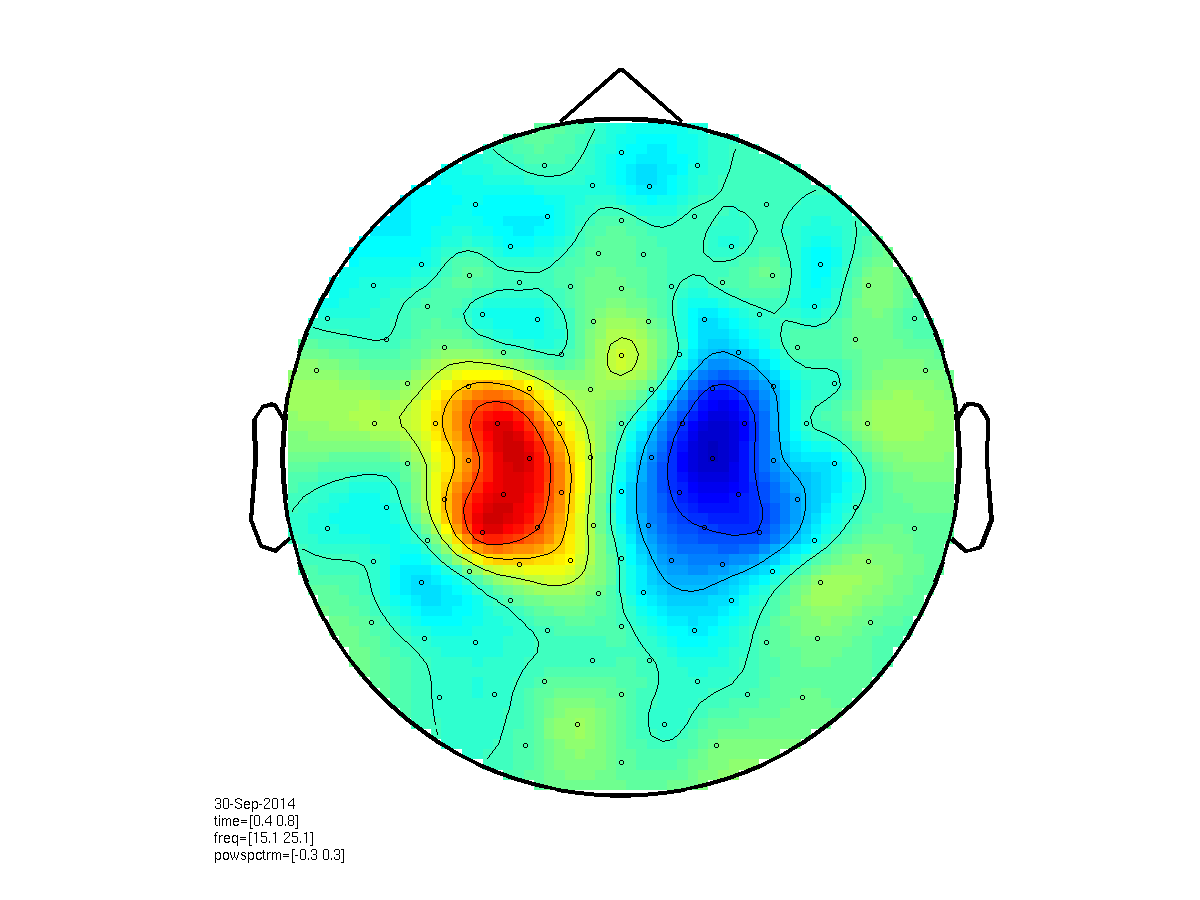Figure: A topographic representation of the time-frequency representations of the difference in beta (15-25 Hz) power, between left and right response, in EEG, after 0.5-1.0s, obtained using ft_topoplotTFR.

Finally, lets take a look at how the topography looks when we use the MEG planar gradiometers.

``````cfg = [];
cfg.baseline     = [-0.5 -0.1];
cfg.baselinetype = 'absolute';
cfg.xlim         = [0.4 0.8];
cfg.ylim         = [15 25];
cfg.zlim         = [-1e-24 1e-24];
cfg.marker       = 'on';
cfg.layout       = 'neuromag306planar.lay';

figure;
ft_topoplotTFR(cfg, TFR_left_MEG);
print -dpng natmeg_freq12.png
``````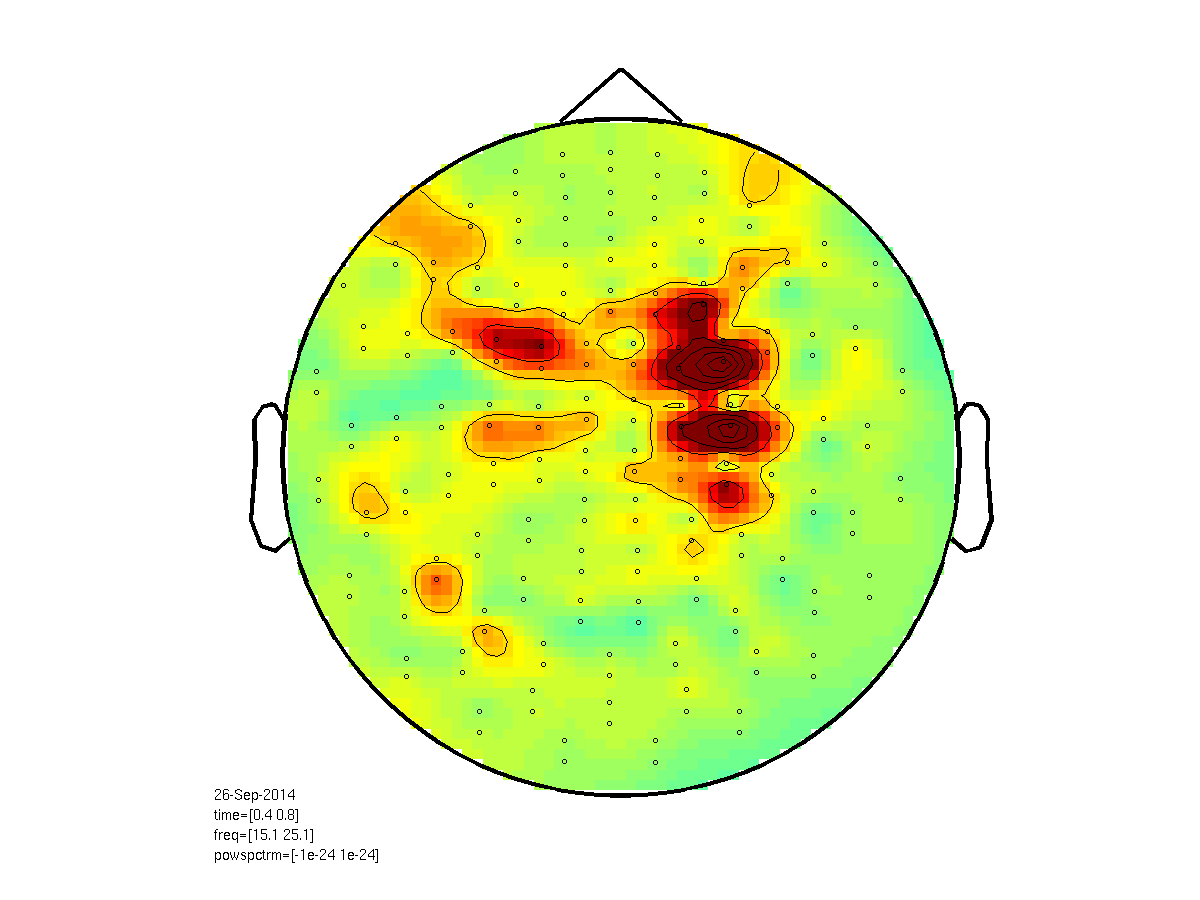Figure: A topographic representation of the time-frequency representations of the relative change in beta (15-25 Hz) power, for gradiometers, after 0.5-1.0s, obtained using ft_topoplotTFR.

Now that looks a bit funky, right? Do you know why?

In fact, we are now plotting the two different gradiometers together. You can see the channel locations being in pairs, one above the other. They are in reality, however, at the same location but oriented differently - radially and axially with respect to the surface of the helmet. They can thereby pick up both radial orientations of the magnetic fields. To use them properly for the purpose of plotting, we should therefor combine them first, adding their fields.

``````TFR_left_MEG_comb  = ft_combineplanar([],TFR_left_MEG);
TFR_right_MEG_comb = ft_combineplanar([],TFR_right_MEG);

cfg = [];
cfg.baseline     = [-0.5 -0.1];
cfg.baselinetype = 'absolute';
cfg.xlim         = [0.4 0.8];
cfg.ylim         = [15 25];
cfg.zlim         = [-4e-24 4e-24];
cfg.marker       = 'on';
cfg.layout       = 'neuromag306cmb.lay';

figure;
ft_topoplotTFR(cfg, TFR_left_MEG_comb);
print -dpng natmeg_freq13.png
``````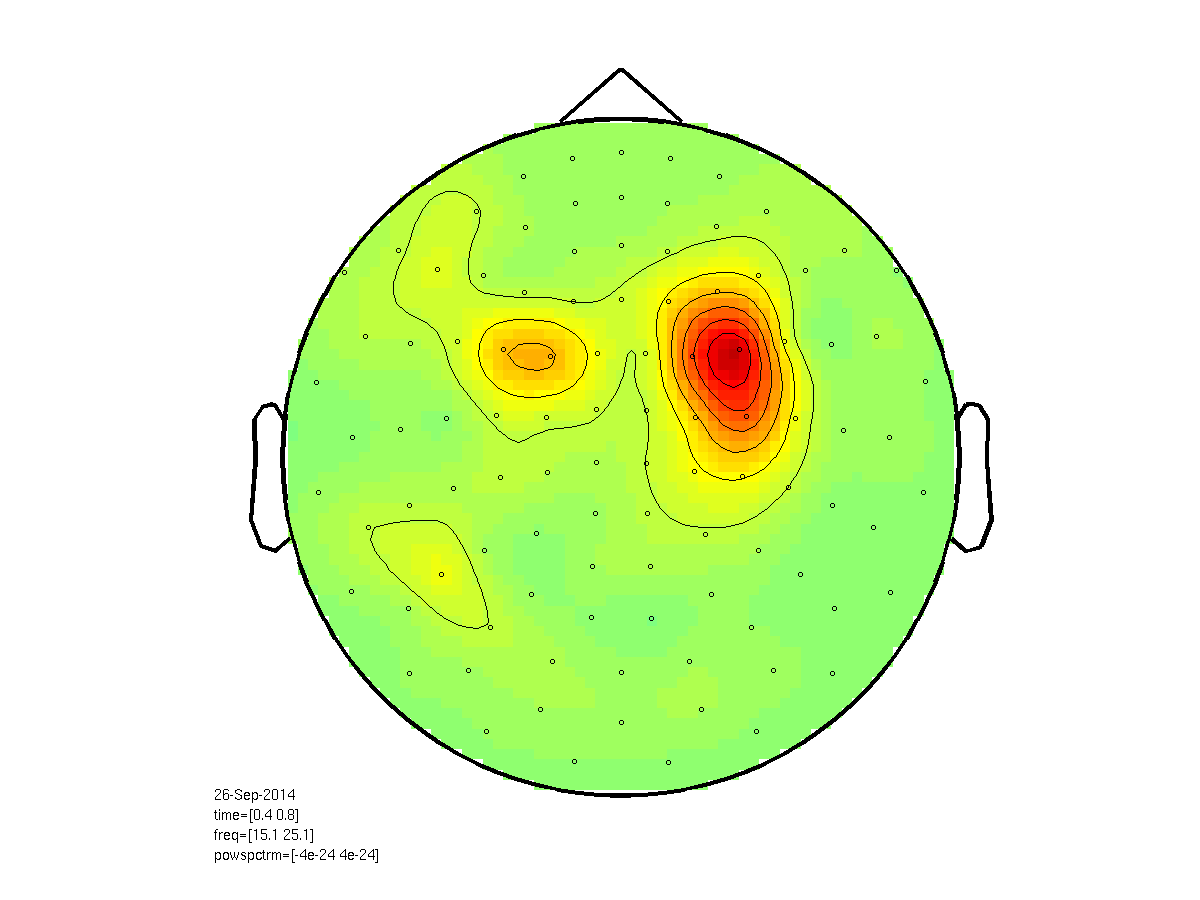Figure: Topographic representation of the time-frequency representations of the relative change in beta (15-25 Hz) power, for combined gradiometers, after 0.5-1.0s, obtained using ft_topoplotTFR.

Now that looks much better!

Finally, let’s plot the difference between conditions using the combined gradiometers. Should we calculate the combination before or after we do the comparison (i.e. (left-right) / (left+right))? Why?

``````cfg = [];
cfg.parameter = 'powspctrm';
cfg.operation = '(x1-x2)/(x1+x2)';

TFR_diff_MEG_comb = ft_math(cfg, TFR_right_MEG_comb, TFR_left_MEG_comb);

cfg = [];
cfg.baseline     = [-0.5 -0.1];
cfg.baselinetype = 'absolute';
cfg.xlim         = [0.4 0.8];
cfg.ylim         = [15 25];
cfg.zlim         = [-0.3 0.3];
cfg.marker       = 'on';
cfg.layout       = 'neuromag306cmb.lay';

figure;
ft_topoplotTFR(cfg, TFR_diff_MEG_comb);
print -dpng natmeg_freq14.png
``````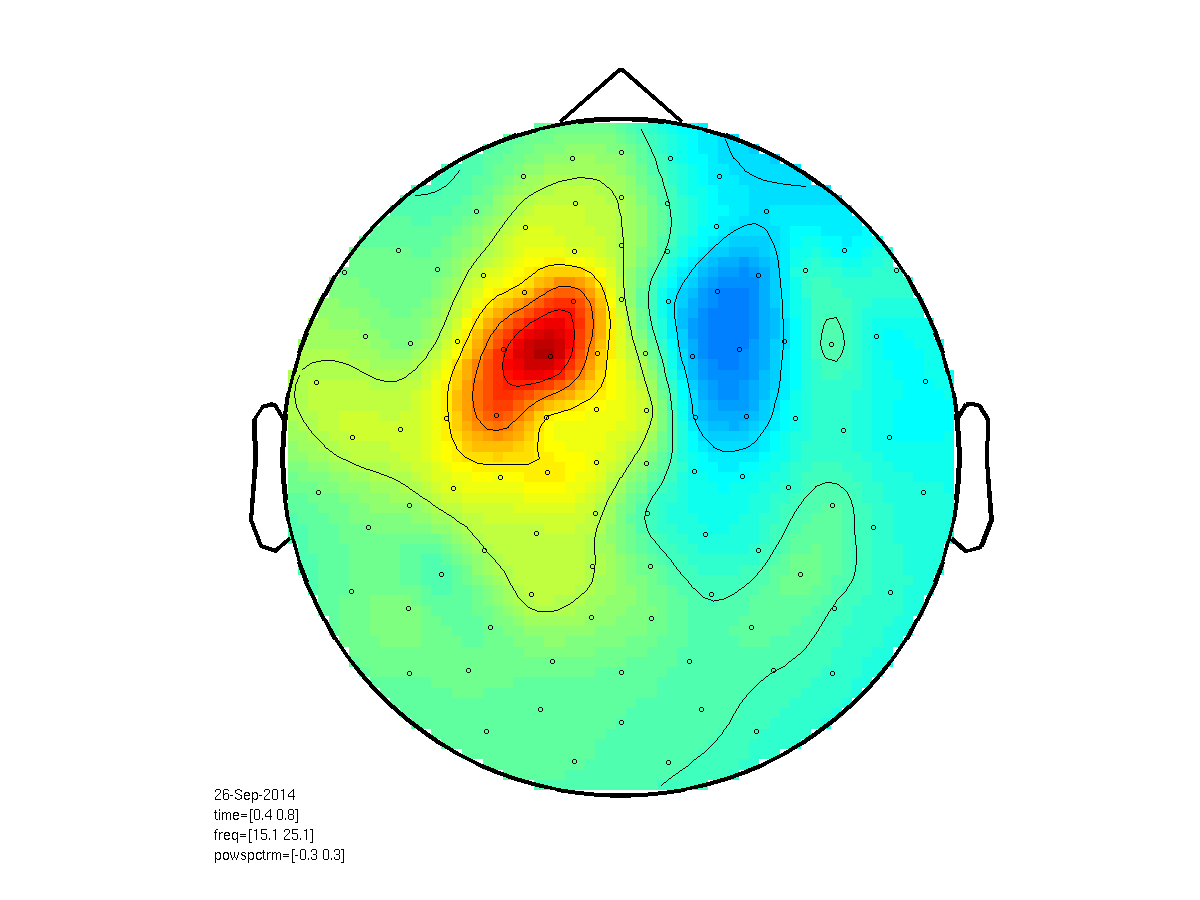Figure: A topographic representation of the time-frequency representations of the differences in beta (15-25 Hz) power, between left and right response, after 0.5-1.0s, obtained using combined planar gradiometers in ft_topoplotTFR.

We have now reached the end of the MEG-EEG part of the tutorial.

Please take some time to reflect on the differences and similarities between EEG and MEG in frequency analysis. Please write down any questions you might have so that we can discuss them together.

## Summary and suggested further reading

This tutorial showed how to do time-frequency analysis on a single’s subject MEG and EEG data and how to plot the time-frequency representations. We took special notice of noticing the differences and similarities between MEG and EEG analysis.

As we noted in the introduction, there are more ways of doing a frequency analysis. If you want to know more about how to do wavelet analysis, adaptive time-windows and multitapers, please take a look at standard (MEG only) time-frequency analysis tutorial, starting at part II. You can copy-paste parts of it into your own script and use them on our dataset.